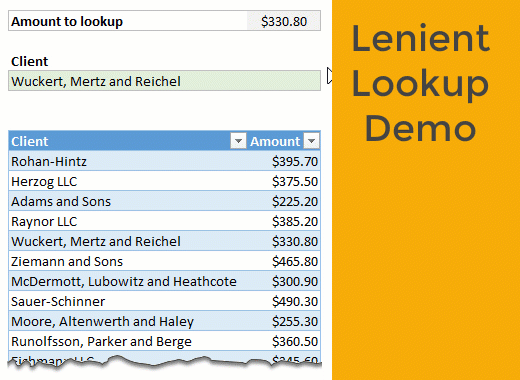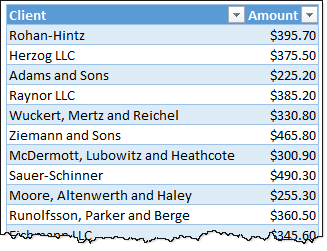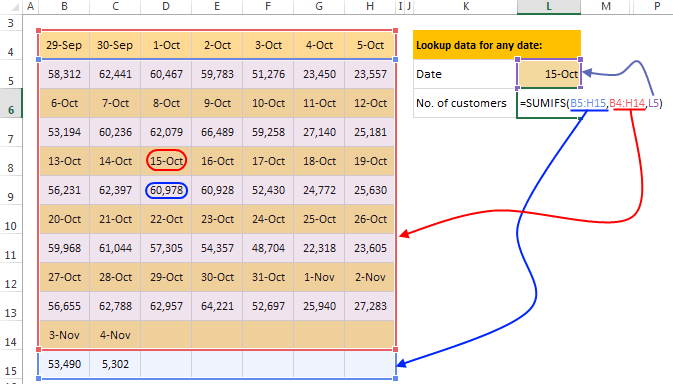# Lenient lookup [Advanced Formula Trick]

Share

We all know VLOOKUP (or INDEX+MATCH) as an indispensable tool in our Excel toolbox. But what if you want the lookups to be a little gentler, nicer and relaxed?

Let’s say you want to lookup the amount \$330.50 against a list of payments. There is no exact match, but if we look 50 cents in either direction, then we can find a match. Here is a demo of what I mean.Unfortunately, you can’t convince VLOOKUP to act nice.

Hey VLOOKUP, I know you are awesome and all, but can you cut me some slack here?

VLOOKUP is tough, reliable and has a cold heart. Or is it?

In this post, let’s learn how to do lenient lookups.

### Data for the problem

Let’s say you have a simple 2 column table like this. Our table is uninspiringly named data.## Lenient lookup – setting up the formula

Our input amount is in cell C3.

Let’s say when looking up for the amount, we want to follow this logic.

1. If an exact match is found, return that
2. Else, see if we can find anything with in 50 cents either side (you can change 50 to whatever you want)
3. If nothing can be found, we want to return “Not found” or similar message

Formulas to use:

1: we can use good old INDEX+MATCH

2: we can use array based INDEX+MATCH

3: we can use IFERROR.

Let’s put everything together.

Our lenient lookup formula (array):

```=IFERROR( INDEX(data[Client], IFERROR(MATCH(\$C\$3,data[Amount],0), MATCH(1, (data[Amount]>(\$C\$3-0.5))*(data[Amount]<(\$C\$3+0.5)),0) )) ,"Not found")```

How does it work?

Let’s go inside out.

MATCH(\$C\$3,data[Amount],0): this formula simply looks for C3 in data[Amount] column and returns the position.

MATCH(1, (data[Amount]>(\$C\$3-0.5))*(data[Amount]<(\$C\$3+0.5)),0): This array formula checks for 1 (TRUE) by looking at data[Amount] between C3-0.5 and C3+0.5

The formula has two Boolean arrays multiplied and it returns a bunch of 1s & 0s.

MATCH then picks up the first such amount.

Inner IFERROR(MATCH(…), MATCH(…)): This acts like a fail-safe switch. If there is no exact match (first one), then lenient match (second one) will be used.

Outer IFERROR(): If no matches are found (exact or lenient) then “Not found” will be printed.

As this is an array formula, you need to press CTRL+Shift+Enter to get the result.

Related material – read these if you have questions about the formula techniques used above:

## Other lenient / almost lookup problems

There are few more variations to this technique. Let’s review them.

Note: all of these are array formulas, so press CTRL+Shift+Enter.

### Ignore decimal portion

We lookup just the whole number portion of the value to find match.

Formula: =INDEX(data[Client], MATCH(G7, INT(data[Amount]),0))

Notes on how it works:

• INT() turns data[Amount] column to whole numbers.
• We then lookup the amount (G7) and return the match

### Amount is at least something, client name begins with S

Formula: =INDEX(data[Client], MATCH(1, (data[Amount]>=G8)*(LEFT(data[Client],1)=”S”),0))

• We use a different Boolean structure with >= and LEFT() formulas. The output will be a bunch of 1s & 0s.
• INDEX+MATCH for find the first such value (G8)

### Closest Amount to input

This is interesting. We use MIN & ABS to find closest amount to input value (G10) and return the client’s name.

Formula: =INDEX(data[Client], MATCH(MIN(ABS(data[Amount]-G10)), ABS(data[Amount]-G10),0))

• ABS(data[Amount]-G10) gives a bunch of absolute (positive) values. The smallest of these will closest to G10.
• MIN() finds the smallest value
• MATCH looks up the minimum value from ABS(data[Amount]-G10)
• INDEX gives corresponding client’s name

### More ways to lookupLookups are an essential part of any data analysis work you do in Excel. Pick up some nifty tricks from these links.

### Got a lookup tip to share?

Have some lookup stories to tell? I am listening. Please post them in comments.

### Get FREE Excel + Power BI Tips

Simple, fun and useful emails, once per week.

Learn & be awesome.

### Welcome to Chandoo.org

Thank you so much for visiting. My aim is to make you awesome in Excel & Power BI. I do this by sharing videos, tips, examples and downloads on this website. There are more than 1,000 pages with all things Excel, Power BI, Dashboards & VBA here. Go ahead and spend few minutes to be AWESOME.

Read my storyFREE Excel tips book

I will be presenting at two events in London in April 2020. Come & join me.

Chandoo is an awesome teacher
5/5

– Jason

Still on fence about Power BI? In this getting started guide, learn what is Power BI, how to get it and how to create your first report from scratch.

### 7 Responses to “Lenient lookup [Advanced Formula Trick]”

1.Excel Gazi Deniz Hoca says:

Hello Dear Excel Guru Chandoo;
First of all, a wholehearted and candid "Happy Birthday" to you venerable mother without whose selfless devotion to her family we Excel lovers would have been deprived of you...

Now, regarding the first leniant lookup example, here is my suggested solution:

=IFERROR(INDEX(data[Client],MATCH(\$C\$3,data[Amount],0)),INDEX(data[Client],MATCH(MIN(ABS(data[Amount]-\$C\$3)),ABS(data[Amount]-\$C\$3),0))&" *")

This solution basically finds THE CLOSEST MATCH to a given lookup value (and the first of such matches in case of multiple occurrences) in a column of UNSORTED VALUES, and then completes the lookup operation upon a different column.

This means my suggested formula would work also when the ABSOLUTE difference between the lookup value and the value of the correct match exceeds 0.50.

The seemingly complicated formula is actually not too much different from yours, Dear Chandoo. The underlying idea also here is to construct an ARRAY OF ABSOLUTE DIFFERENCES and then to figure out the [first] position of the MINIMUM OF SUCH DIFFERENCES in the very same array.

In the event of an EXACT MATCH, the first INDEX() function inside the IFERROR() would return the lookup value of that exact match without appending the "*" character to the result. Otherwise, the second INDEX() function would be triggered and an " *" would be appended to the outcome of the IFERROR().

Hope this solution proves helpful when one needs to perform a lookup operation with APPROXIMATE MATCH upon an array of UNSORTED VALUES.

Cheers'n greetings from Istanbul. --- Deniz

2.Veeru says:

Hi,

Little confuse here.....what does data[Client] and data[Amount] stand for..

I guess data[Client] is whole data for a particular sheet but what about data[Amount] is this Array of amount column??

3.David N says:

Don't forget you can use a helper INDEX, as well as AGGREGATE for the "Closest Amount" problem, to make any of these possible without a CSE array.

Within \$0.50
=INDEX(data[Client],MATCH(1,INDEX(--(ABS(data[Amount]-\$C\$3)=\$G\$8)*(LEFT(data[Client],1)="S"),0),0))

Between 300 and 350 and not an LLC
=INDEX(data[Client],MATCH(1,INDEX((data[Amount]>=300)*(data[Amount]<=350)*(RIGHT(data[Client],3)"LLC"),0),0))

Closest amount
=INDEX(data[Client],MATCH(AGGREGATE(15,4,ROUND(ABS(\$G\$10-data[Amount]),2),1),INDEX(ROUND(ABS(\$G\$10-data[Amount]),2),0),0))

•David N says:

Not sure what happened to my last post, the less/greater symbols I think, but the middle vanished. So trying one more time.

Within \$0.50
=INDEX(data[Client],MATCH(1,INDEX(--(ABS(data[Amount]-\$C\$3)<=0.5),0),0))

Ignore decimals
=INDEX(data[Client],MATCH(\$G\$7,INDEX(TRUNC(data[Amount],0),0),0))

At least amount and begins with S
=INDEX(data[Client],MATCH(1,INDEX((data[Amount]>=\$G\$8)*(LEFT(data[Client],1)="S"),0),0))

Between 300 and 350 and not an LLC
=INDEX(data[Client],MATCH(1,INDEX((data[Amount]>=300)*(data[Amount]<=350)*(RIGHT(data[Client],3)<>"LLC"),0),0))

Closest amount
=INDEX(data[Client],MATCH(AGGREGATE(15,4,ROUND(ABS(\$G\$10-data[Amount]),2),1),INDEX(ROUND(ABS(\$G\$10-data[Amount]),2),0),0))

4.MF says:

Hi Chandoo,
This is one of my lookup stories... it's about looking up partially match records.
https://wmfexcel.com/2014/01/23/how-to-lookup-only-partially-matched-value-crazy-lookup/

Cheers,
MF

5.Abhishek says:

This advanced excel tut is amazing and I have learned a lot from this. I am bookmarking this page for my future reference. Thanks for creating such a wonderful articles. 🙂

### Get FREE Excel & Power-BI Newsletter

One email per week with Excel and Power BI goodness. Join 100,000+ others and get it free.